## When Your Formula Doesn’t Work: Formula Parse Errors in Google Sheets

Whether you’re just starting out with Google Sheets or are a seasoned pro, sooner or later one of your formulas will give you a formula parse error message rather than the result you want.

It can be frustrating, especially if it’s a longer formula where the formula parse error may not be obvious.

In this post, I’ll explain what a Google Sheets formula parse error is, how to identify what’s causing the problem, and how to fix it.

## What is a formula parse error?

Before we get into the different types of errors, you might be wondering what does formula parse error mean?

Essentially, it means Google Sheets can’t interpret your formula. It can’t fulfill the formula request so it returns an error message.

There are a variety of ways this can happen — everything from typos to mathematical impossibilities — and we’ll explore them all in detail below.

Understanding the meaning behind the error messages, and learning how to fix them, is a crucial step to becoming a formula pro in Google Sheets.

## Auditing and Debugging Formula Parse Errors in Google Sheets

Match the error message in your Google Sheet to the sections below, and find out what might be causing your error.

Here’s a Google Sheet with all these examples in.

### 1. A formula parse error message popup prevents me entering my formula

You think you’ve finished your formula, so you hit enter and boom! You get slapped with a popup message box `"Houston, we have a problem"` or similar: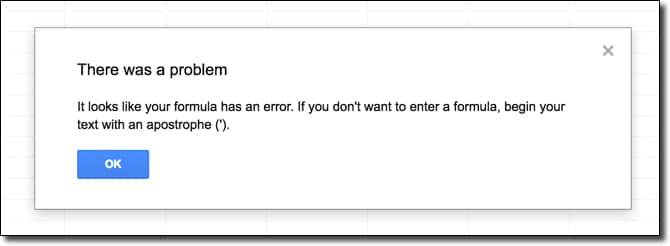It’s reasonably rare that you’ll experience this, and it usually points to some fundamental problem with your formula.

For example, imagine that as you hit the Enter key, you also accidentally struck the “\” key (which is right above the Enter key) and inadvertently added that to the end of your formula: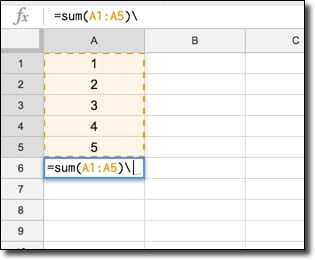This will result in the popup error message. It’s easily corrected by removing the unwanted character.

#### How to correct this error?

Try to avoid these in the first place by checking your formula prior to hitting enter. Make sure you’re not missing a cell reference and you don’t have any unwanted characters lurking.

### 2. I’m getting an #N/A error message. How do I fix it?

The #N/A formula parse error signifies that a value is not available.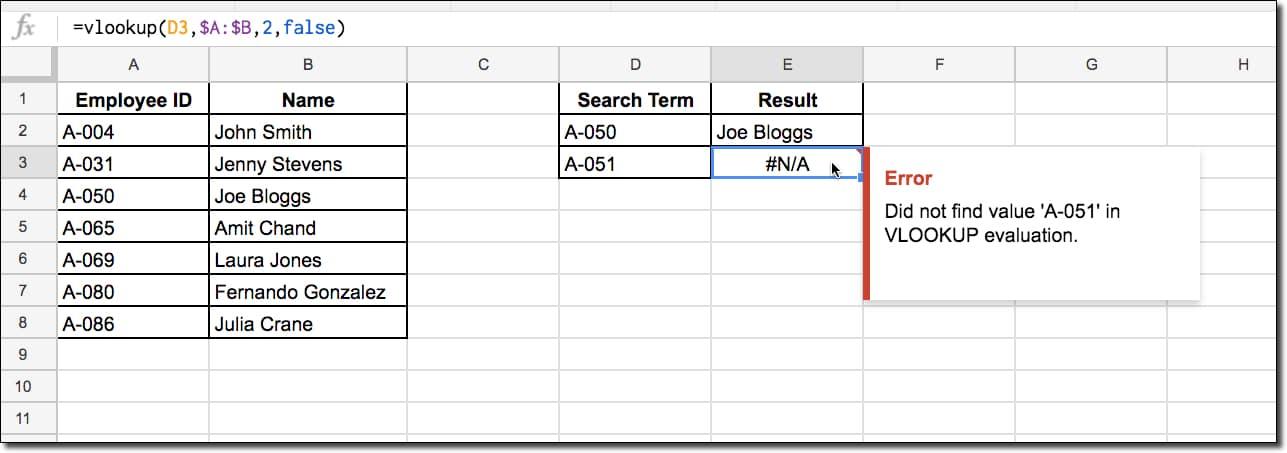It happens most frequently when you’re using a lookup formula (e.g. VLOOKUP) and the search term isn’t found. This is exactly what has happened in the exact match VLOOKUP in the image above. The search term A-051 is not in our data table so the formula returns #N/A.

This formula is not wrong or broken, so we don’t want to delete it. However, it would be cool if you could display a custom message, something like “Result not found”, instead of #N/A error message, especially if you have a lot of these errors showing. It gives the spreadsheet user much more information and reduces confusion.

Thankfully we can:

#### How to correct an #N/A error?

Well, there’s this super handy formula IFERROR:

=IFERROR(original formula, value to display if the original formula gives an error)

In this VLOOKUP example, the full formula would look like this:

as shown in this example: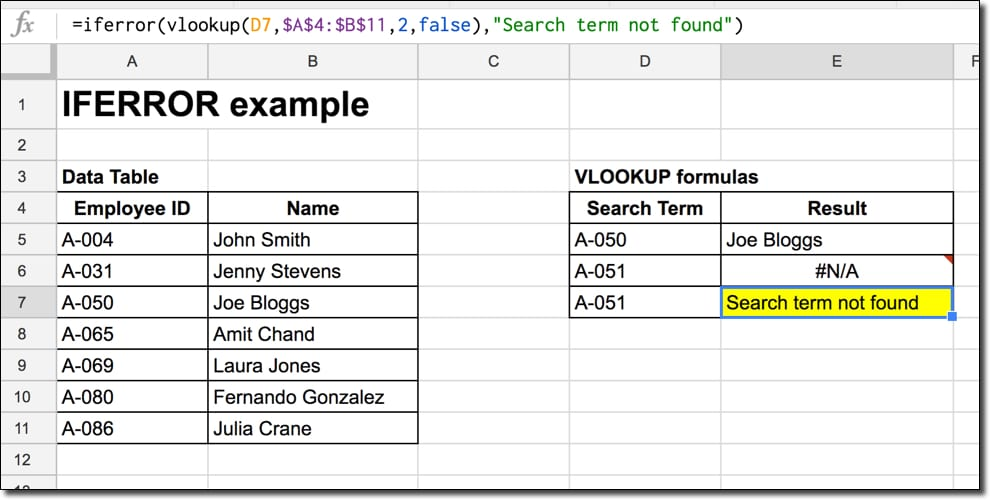### 3. I’m getting an #DIV/0! error message

This formula parse error happens when a number is divided by zero, which can occur when you have a zero or a blank cell reference in the denominator.

In layman’s terms, what this means is that we trying to compute something like this:

= A / 0

which has no meaning (because there is no answer, which, when multiplied by 0, would return A).

Read more about division by 0 here, although it gets super technical super quickly.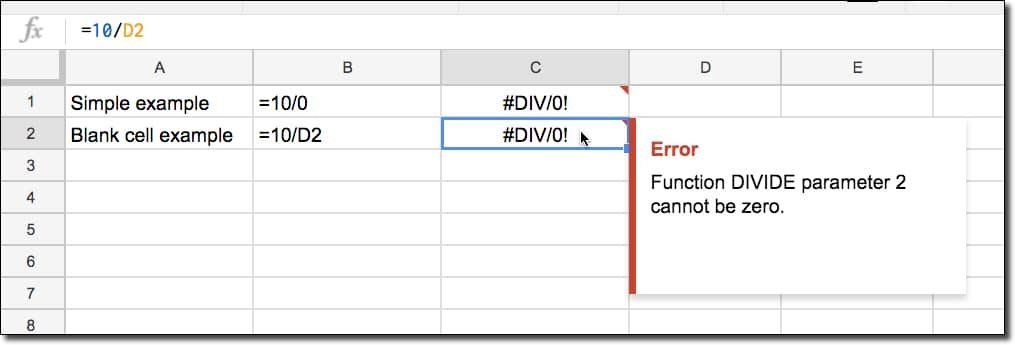Another example is using a formula like AVERAGE with a blank range.

So, = AVERAGE(A1:A10)   will cause a #DIV/0! error if the range A1:A10 contains no numerical values.

#### How to correct an #DIV/0! error?

Well the first thing to do is determine why your denominator is evaluating to zero.

You can select the denominator and see what it is evaluating to by highlighting it in the formula bar, and seeing what the result is in the little popup box, as shown in this image: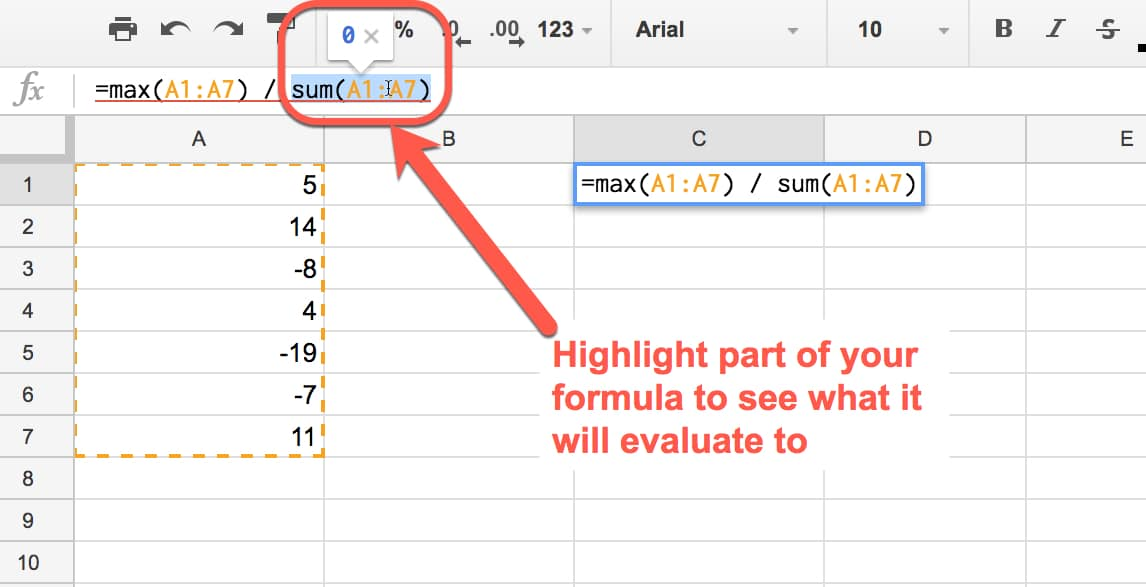In this case, the formula in the denominator SUM(A1:A7) evaluates to 0, which causes the error. So check whether your denominator result is 0.

Next, check whether you have linked to blank cells or a blank range in your denominator. Then you can either fill in the blank cell or range, or select a different cell or range for your formula.

If your formula is correct and your cell/ranges are not unintentionally blank, then you’ll want to handle the #DIV/0! error. It looks unsightly and makes your spreadsheet look unfinished if you leave these errors floating around.

As with the #N/A error example, use the IFERROR formula to wrap your current formula and specify a result for when a #DIV/0! error occurs. You might want to output an error message, e.g. “Division by 0 error”, or maybe a specific value, e.g. 0: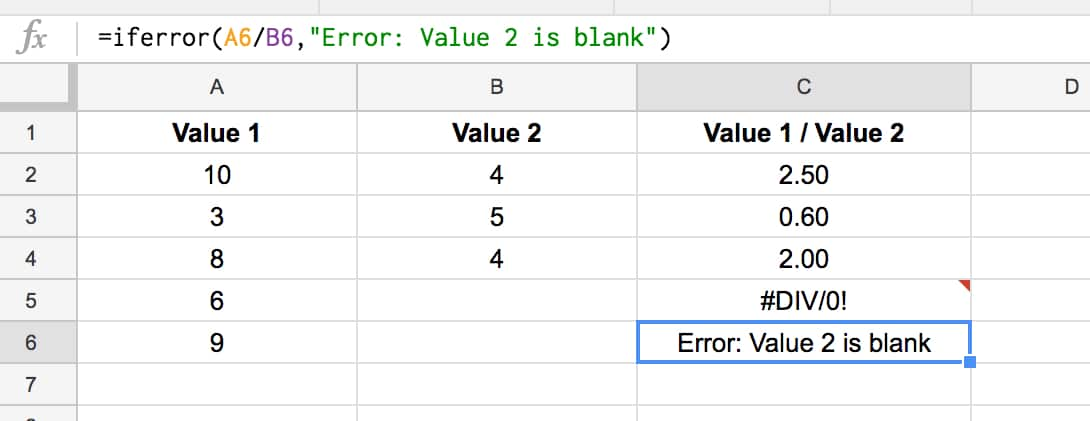### 4. I’m getting an #VALUE! error message

This formula parse error typically occurs when your formula is expecting a certain data type as an input but receives the wrong type, for example trying to do math operations on a text value instead of a numerical value.

Spaces in your cells can also cause this error message.

In this example, cell B1 contains a space, which is a string value and causes the #VALUE! error because Google Sheets can’t perform a math operation on it, as seen in this error message: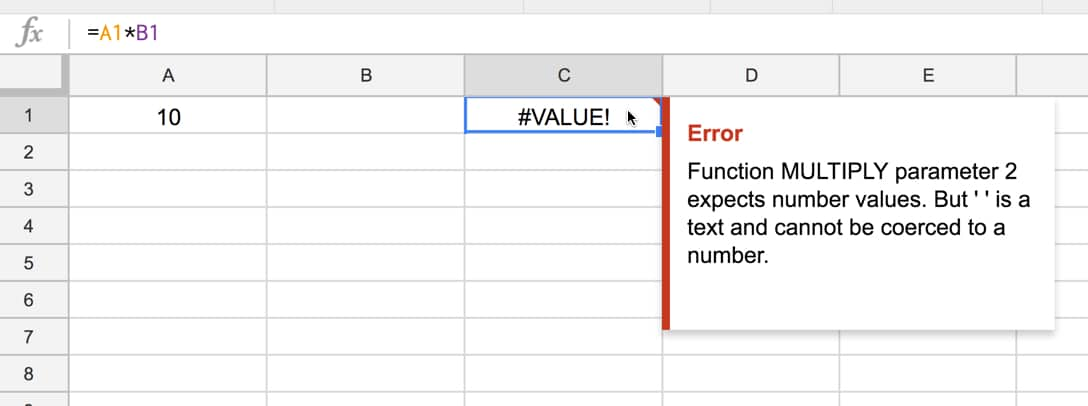In general, Google Sheets do a pretty good job of coercing text into numbers when needed. If you enter a value into a cell with some spaces, format it as text and then try to do math on it, Google Sheets will actually force the text into a number and still perform the calculation.

Another cause of #VALUE! errors is mixing US and Rest of World date formats.

US dates have the form MM/DD/YYYY whilst the Rest of the World goes for DD/MM/YYYY. If you have a mix of the two and try to subtract them to get the number of days between them for example, you’ll get the #VALUE! error.

(In fact, it’s the same text/number issue happening underneath the surface. Dates are stored as numbers, but if you’re date is in the wrong format for the country setting for your spreadsheet, it’ll be stored as a text string and Google won’t know it’s meant to be a date.)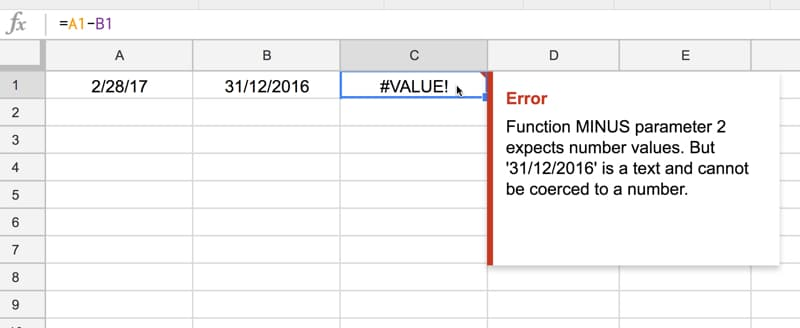Here the correct answer should have been 59, the number of days between the 28 Feb 2017 and the 31st Dec 2016.

#### How to correct an #VALUE! error?

The error message should give you some information on which part of your formula is causing the problem.

Search for any possible text/number mismatches, or cells containing errant spaces. If you click into a cell and the flashing cursor has a gap between itself and the element it’s next to, then you’ll have a space there.

Cells can look empty but still contain spaces: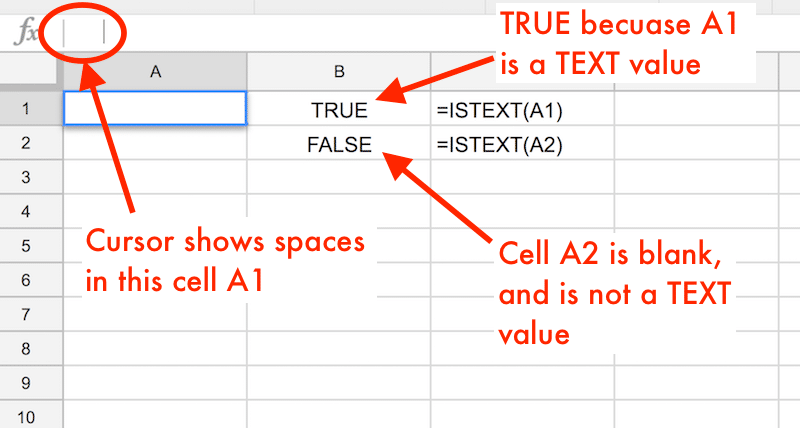Dates with spaces in the middle won’t work either: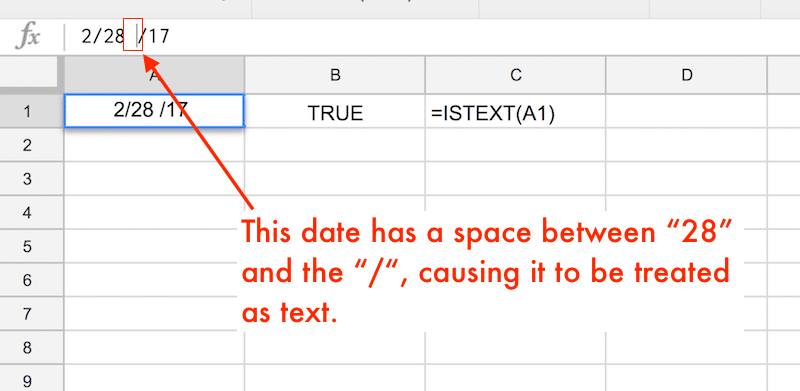### 5. I’m getting a #REF! error message

The #REF! formula parse error occurs when you have an invalid reference.

Missing reference: For example when you reference a cell in your formula that has since been deleted (not the value inside the cell, but the whole cell has been deleted, typically when you’ve deleted a row or column in your worksheet).

In this example, the original formula was = A1 * B1, but when I deleted column A, the formula went haywire because of the missing reference: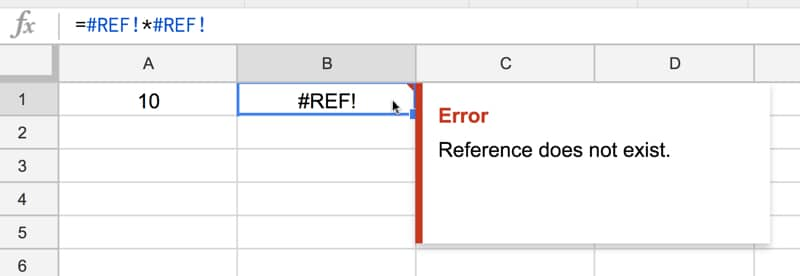Another way that a formula can refer to missing references is when you copy a formula with a relative range at the edge of your sheet. When you copy and paste, it’s possible the relative range moves as if it were outside the bounds of the sheet, which is not allowed and will cause a #REF! error.

In this example, the sum function adds the cells in the 3 rows above. When I try to copy-paste the sum function into a new cell with fewer than 3 rows above, it’ll give me the #REF! error: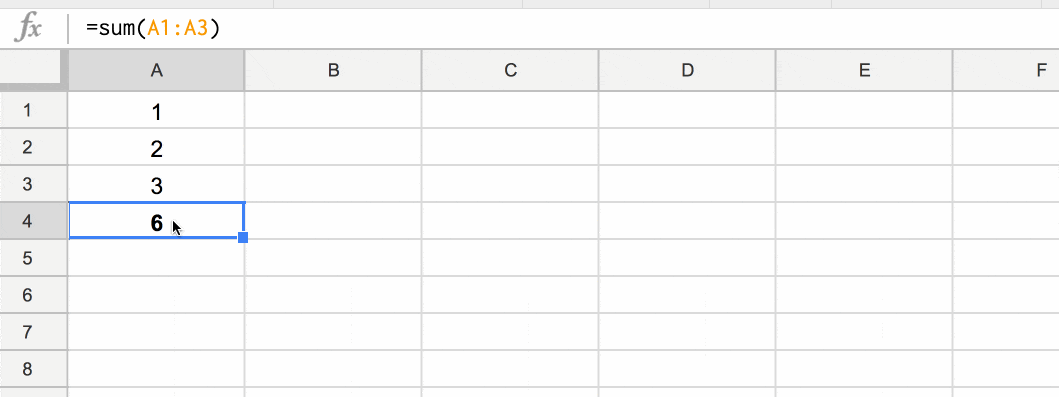Lookup out of bounds: You’ve probably seen the #REF! error if you use lookup formulas frequently, when you’ve tried to return a value outside of ranges you’ve specified. In this VLOOKUP example, I’m trying to return an answer from the 3rd column of a search table that only has 2 columns: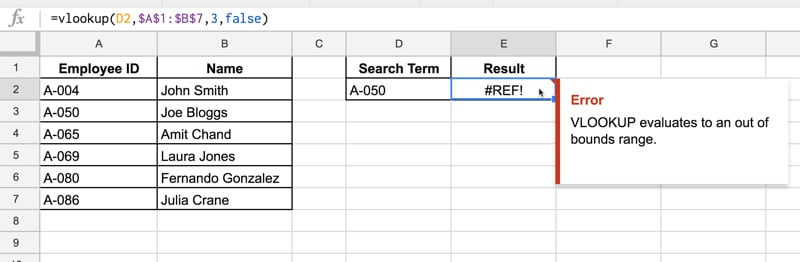Circular dependency: You’ll also get a #REF! error when a circular dependency is detected (when the formula refers to itself).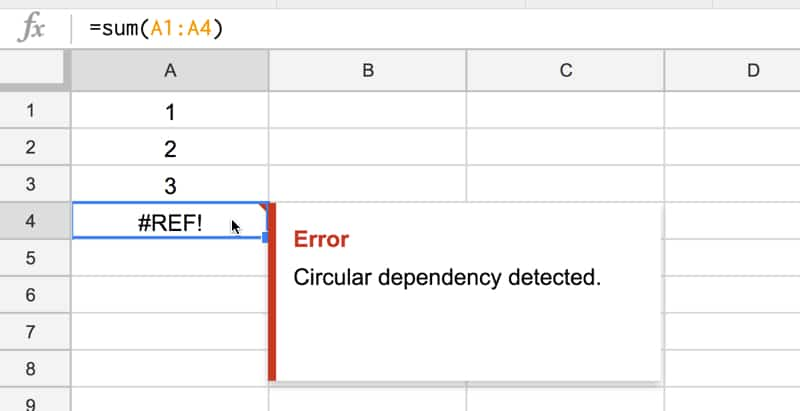In this example, I have numbers in the range A1 to A3, but the SUM formula in cell A4 tries to sum from A1 to A4, which includes itself. Hence, we have a circular argument where cell A4 is trying to be both an input and output cell, which is not allowed.

#### How to correct a #REF! error?

First of all, read the error message to determine what kind of #REF! error you’re dealing with. This should give you a big hint on how to correct the error.

For deleted references, look for the #REF! error is inside your formula, and replace the #REF! with the correct reference to a cell or range.

For out-of-bound lookup errors, look through your formula carefully and check your range sizes against any row or column indexes you’re using.

For circular dependencies, find the reference that’s causing the problem (i.e. where you refer to the current cell inside your formula too) and modify it.

### 6. I’m getting a #NAME? error message

The #NAME? formula parse error signifies a problem with your formula syntax.

The most common reason for this error is a misspelling in one of your function names.

In this example, I misspelt the SUM function as SUMM, which Google Sheets didn’t recognize, so returned an error: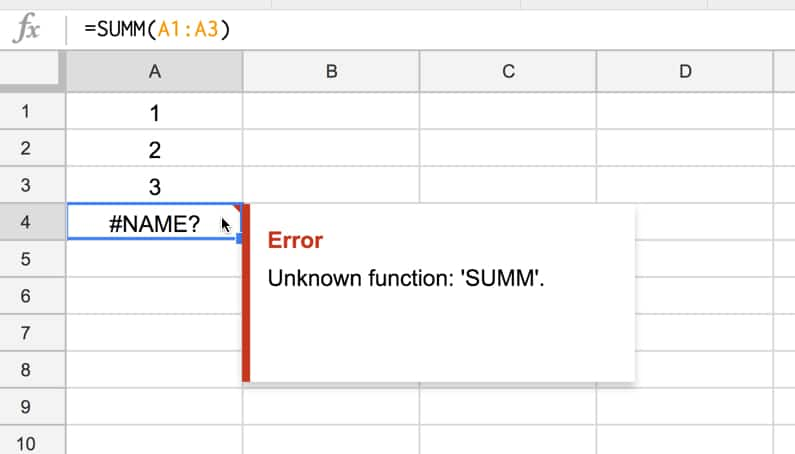Another reason for a #NAME? error is referencing a named range which doesn’t actually exist, or is misspelt.

So

=SUM(profit)

will give you a #NAME? error if the named range profit does not exist

Missing quotation marks around a text value, as shown in this simple formula, will also cause a #NAME? error:

=CONCAT(“First”,Second)

(The word Second is missing quotation marks.)

#### How to correct an #NAME? error?

Check your function names are correct. Use the function helper wizard to reduce the chances of errors happening, especially for the functions with longer names. As you start typing your formula, you’ll see a menu of functions, which you can select with the up and down arrows and Tab.

Check you have defined all named ranges before using them in your formulas and that they all have the correct spellings.

Check any text values are entered with the required quotation marks.

Lastly, have you missed the colon in your range references? It’ll be obvious because it won’t be highlighted correctly.

This formula =SUM(A1A10)

is missing the colon between A1 and A10 and will throw a #NAME? error.

It should of course read =SUM(A1:A10)

### 7. I’m getting an #NUM! error message

The #NUM! formula parse error is shown when your formula contains numeric values that aren’t valid.

The classic example is trying to find the square root of a negative number, which isn’t allowed: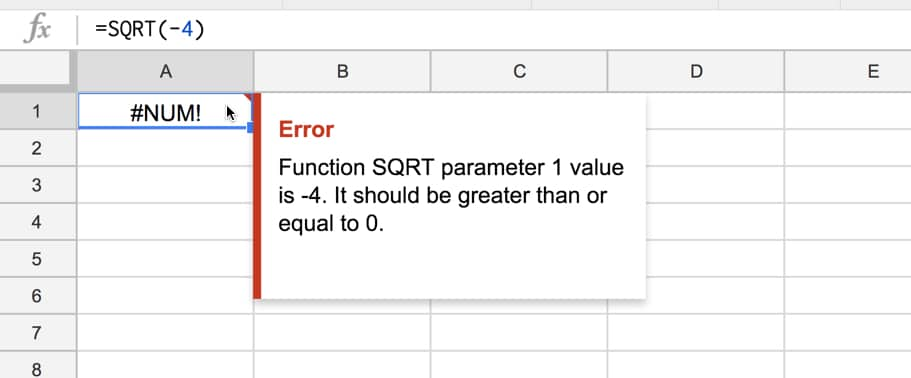(For any math geeks out there, you’ll know that you can resolve square roots of negative numbers with imaginary numbers, but these are outside the realm of spreadsheets.)

Some other functions that can result in #NUM! error messages are the SMALL and LARGE functions. If you try to find the smallest n-th value in your dataset, where n is outside the count of values in your dataset, you’ll get a #NUM! error.

For example, you ask Google Sheets to find the 10th smallest number in a dataset that only has 5 values in it: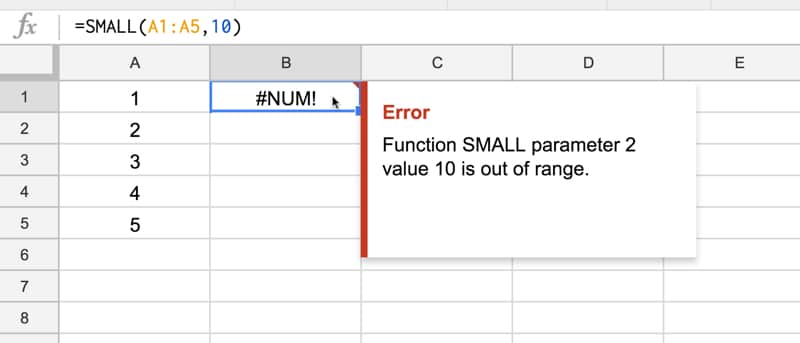(Why this doesn’t return a #REF! error like the VLOOKUP out of bounds example, I don’t know.)

#### How to correct a #NUM! error?

You need to check the numeric arguments in your formula. The error message should give you some hints about which part of the formula is causing the issue.

### 8. I’m getting an #ERROR! formula parse error message

This formula parse error message is unique to Google Sheets and doesn’t have a direct equivalent in Excel. It means that Google Sheets can’t understand the formula you’ve entered, because it can’t parse the formula to execute it.

For example, if you manually type in a \$ symbol to refer to an amount, but Google Sheets thinks you’re referring to an absolute reference: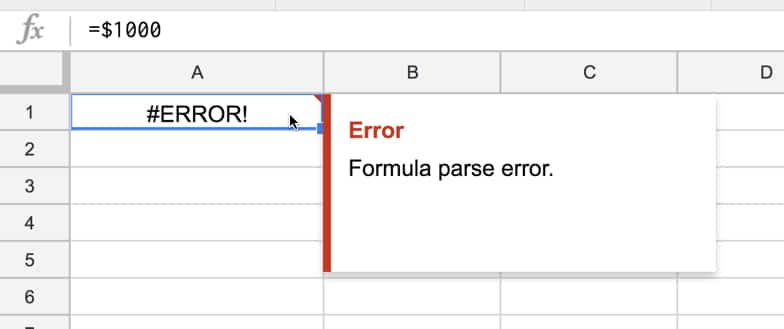or you’ve missed a “&” when concatenating text and numerical values: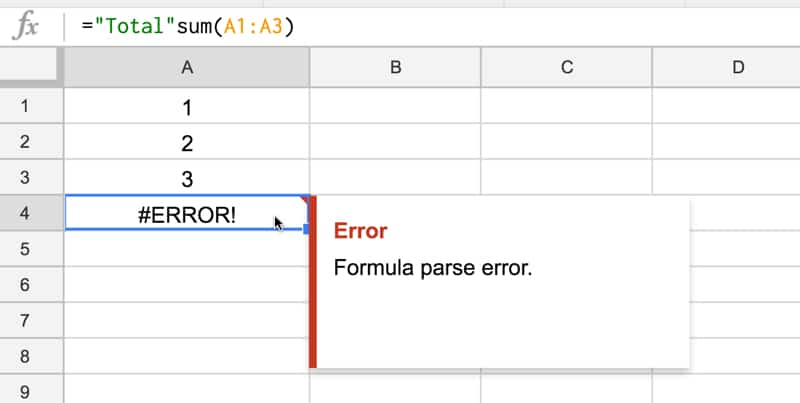In this case the formula should be: =”Total “&sum(A1:A3)

Another case, caused when we messed up the closing brackets of a formula: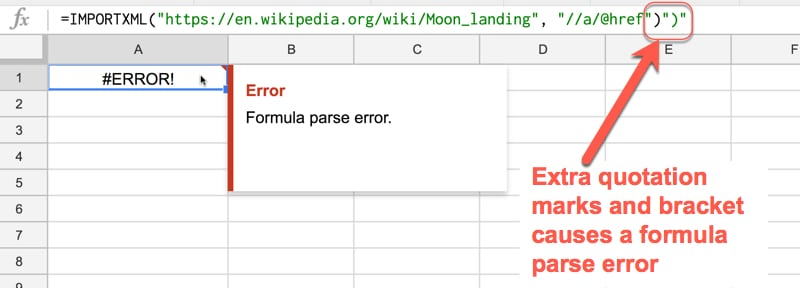#### How to correct an #ERROR! error?

Carefully check your formula for accuracy.

You want to ensure you’ve got the correct number of brackets and correct join syntax between text and numerical values (e.g. using “&”).

When you want to show values with currency symbols or as percentages, don’t manually type in the “\$” or the “%”. Instead enter a plain number and then use the formatting options to change it to the style you want.

### 9. I’m getting an #NULL! error message

I haven’t been able to recreate a #NULL! formula parse error in the wild but theoretically, it exists!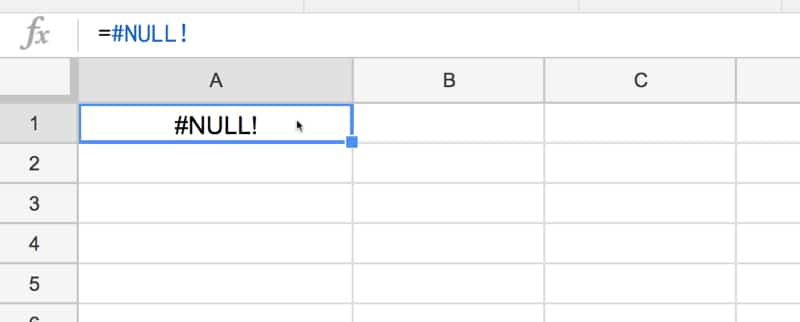(If you have one showing in your sheet, let me know! I’d love to update this article with an example here.)

### 10. Other strategies for dealing with a formula parse error

Look for red highlighting in your formula as this will help identify the source of your error e.g. in the case of too many brackets, the extra, superfluous ones will be highlighted in red.

Peeling back the onion: this is a technique to debug errors for long, complex formulas. Unwrap the outer functions in your formula one-by-one, until you get it working again. Then you can start to add them back one-by-one again, and see exactly which step is causing the issue and fix that.

Different syntax in different countries: Some European countries will use semi-colons “;” in place of commas “,” so this could be a cause of your error. Compare these two formula, which have identical inputs and outputs, but the syntax is different for users in different countries (locales).

=ArrayFormula(VLOOKUP(A1;Sheet2!A:I;{2\3\4\5\6\7\8};FALSE))

is the same formula as this:

=ArrayFormula(VLOOKUP(A1,Sheet2!A:I,{2,3,4,5,6,7,8};FALSE))

#### Pro tip:

Use apostrophe at the start of a formula to turn it into a text string, which won’t execute. This is sometimes useful for seeing your whole formula for debugging, keeping a copy of your formula so you can copy and paste bits of it elsewhere for testing.

### 11. Functions to help deal with formula parse errors in Google Sheets

A few other functions related to formula parse errors are worth knowing about.

In fact, there is even a function to generate #N/A errors. It’s of limited use, but can be helpful for doing data validation in more complex formulas.

=NA()    will output an #N/A error. (Google Docs Help on NA)

=ERROR.TYPE(value)    will return a number corresponding to the error type:

• 1 for #NULL!
• 2 for #DIV/0!
• 3 for #VALUE!
• 4 for #REF!
• 5 for #NAME?
• 6 for #NUM!
• 7 for #N/A
• 8 for all other errors

=ISNA(value)
checks whether a value is the error #N/A, and will give the output TRUE for a #N/A error and FALSE otherwise. (Google Docs Help on ISNA)

=ISERR(value)
checks whether a value is any error other than the #N/A error. (Google Docs Help on ISERR)

=ISERROR(value)
checks whether a value is an error, and will give the output TRUE for any error. (Google Docs Help on ISERROR)

These functions can be summarized in the following table: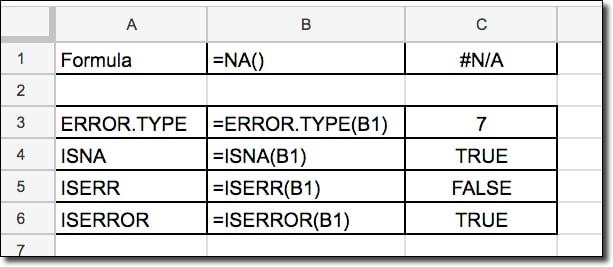## 13. Help! My formula is STILL not working

Take a deep breath, don’t panic! There’s an army of Google Sheets super users out there who would love to help you fix your issue, free of charge, in the active help forums.